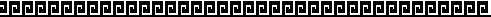Monte Carlo Value at Risk

An Excel-Sheet that calculates the VAR for a portfolio of three riskfactors for an one day horizon. The calculation follows the ideas provided by the Technical Documents of RiskMetrics.
Input parameters are: Covariance matrix of rel. returns, start value of each asset, number of assets per type, number of simulations and the confidence level. In a second sheet the covariance matrix can be estimated from historical prices.
A detailed describtion of the Sheet will follow soon.